# How does SVM works?

Linear SVM:

The working of the SVM algorithm can be understood by using an example. Suppose we have a dataset that has two tags (green and blue), and the dataset has two features x1 and x2. We want a classifier that can classify the pair(x1, x2) of coordinates in either green or blue. Consider the below image: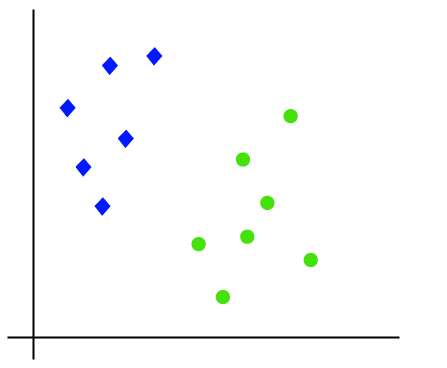So as it is 2-d space so by just using a straight line, we can easily separate these two classes. But there can be multiple lines that can separate these classes. Consider the below image: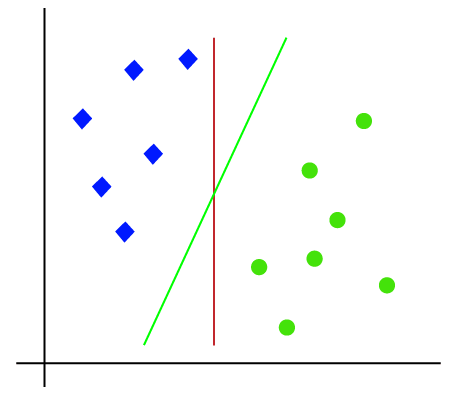Hence, the SVM algorithm helps to find the best line or decision boundary; this best boundary or region is called as a hyperplane . SVM algorithm finds the closest point of the lines from both the classes. These points are called support vectors. The distance between the vectors and the hyperplane is called as margin . And the goal of SVM is to maximize this margin. The hyperplane with maximum margin is called the optimal hyperplane .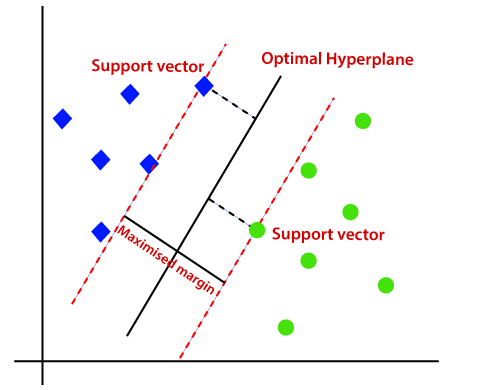Non-Linear SVM:

If data is linearly arranged, then we can separate it by using a straight line, but for non-linear data, we cannot draw a single straight line. Consider the below image: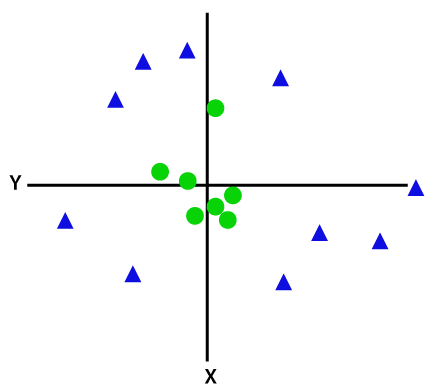So to separate these data points, we need to add one more dimension. For linear data, we have used two dimensions x and y, so for non-linear data, we will add a third dimension z. It can be calculated as:

z=x2 +y2

By adding the third dimension, the sample space will become as below image: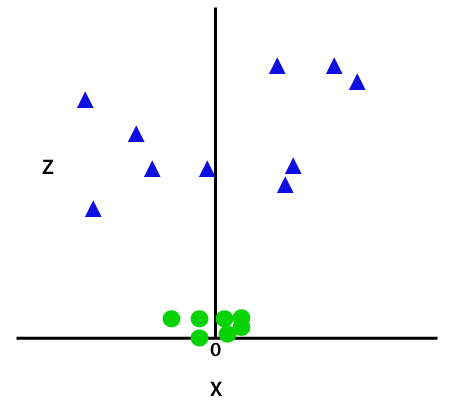So now, SVM will divide the datasets into classes in the following way. Consider the below image: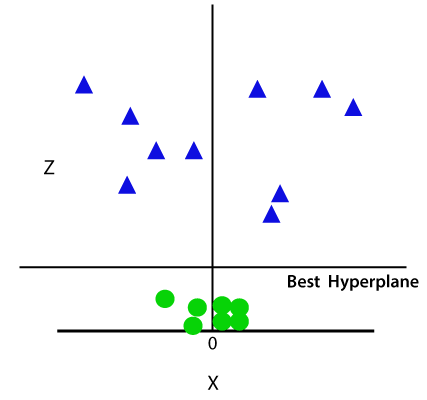Since we are in 3-d Space, hence it is looking like a plane parallel to the x-axis. If we convert it in 2d space with z=1, then it will become as: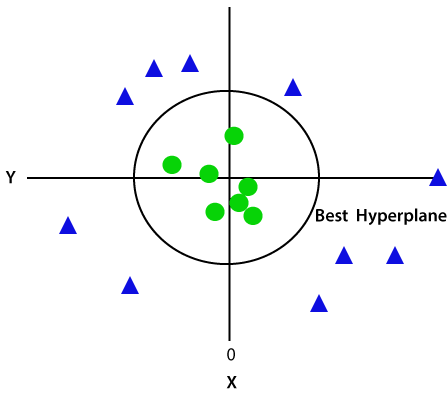Hence we get a circumference of radius 1 in case of non-linear data.